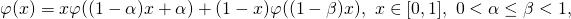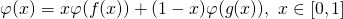# On the stability of two functional equations arising in mathematical biology and theory of learning

### Full PDFcreative_2019_28_1_91_95

In this paper, we prove the Hyers-Ulam stability and the
Hyers-Ulam-Rassias stability of the following two functional equationsandwhich is an open problem raised by Berinde and Khan [Berinde, V. and Khan, A. R., On a functional equation
arising in mathematical biology and theory of learning, Creat. Math. Inform., 24 (2015), No. 1, 9–16].